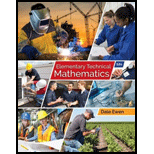# Solve Exercises 27-30 graphically: The sum of two resistances is 14 Ω . Their difference is 6 Ω . Find the two resistances. If we let R 1 and R 2 be the two resistances, the equation is R 1 + R 2 = 14 R 1 − R 2 = 6### Elementary Technical Mathematics

12th Edition
Dale Ewen
Publisher: Cengage Learning
ISBN: 9781337630580

#### Solutions

Chapter
Section### Elementary Technical Mathematics

12th Edition
Dale Ewen
Publisher: Cengage Learning
ISBN: 9781337630580
Chapter 9.1, Problem 27E
Textbook Problem
1 views

## Solve Exercises 27-30 graphically:The sum of two resistances is 14 Ω . Their difference is 6 Ω . Find the two resistances. If we let R 1 and R 2 be the two resistances, the equation is R 1 + R 2 = 14 R 1 − R 2 = 6

To determine

To calculate: The value of two resistances R1 and R2 whose sum is 14Ω and the difference is 6Ω.

### Explanation of Solution

Given Information:

The provided equations are,

R1+R2=14,R1R2=6

Calculation:

Consider the provided equations,

R1+R2=14 ...... (1)

And,

R1R2=6 ...... (2)

Solve the equation (1) for R2,

R2=14R1 ...... (3)

Find three solutions for the equation R2=14R1,

R1R2=14R1R24144106146881486

The solutions for the first equation in the form of (R1,R2) are (4,10),(6,8),(8,6).

Plot these three points on the graph and connect them with a line as follows,

Now, solve the second equation for R2,

R1R2=6R2=6R1

Or,

R2=R16 .....

### Still sussing out bartleby?

Check out a sample textbook solution.

See a sample solution

#### The Solution to Your Study Problems

Bartleby provides explanations to thousands of textbook problems written by our experts, many with advanced degrees!

Get Started

Find more solutions based on key concepts
In problems 15-22, simplify by combining like terms. 15.

Mathematical Applications for the Management, Life, and Social Sciences

Sketch the graphs of the equations in Exercises 512. xy=4

Finite Mathematics and Applied Calculus (MindTap Course List)

POPULATION Growth A major corporation is building a 4325-acre complex of homes, offices, stores, schools, and c...

Applied Calculus for the Managerial, Life, and Social Sciences: A Brief Approach

Find the distance between the points (-2, 1) and (2, 4).

Calculus: An Applied Approach (MindTap Course List)

For

Study Guide for Stewart's Multivariable Calculus, 8th

Evaluate 11(x2x3)dx a) 32 b) 56 c) 12 d) 23

Study Guide for Stewart's Single Variable Calculus: Early Transcendentals, 8th

Fill in the blanks. logbMN= logbM________logbN

College Algebra (MindTap Course List)